# ISEE Middle Level Math : Data Analysis and Probability

## Example Questions

← Previous 1 3 4 5 6 7 8 9 57 58

### Example Question #1 : How To Use A Venn Diagram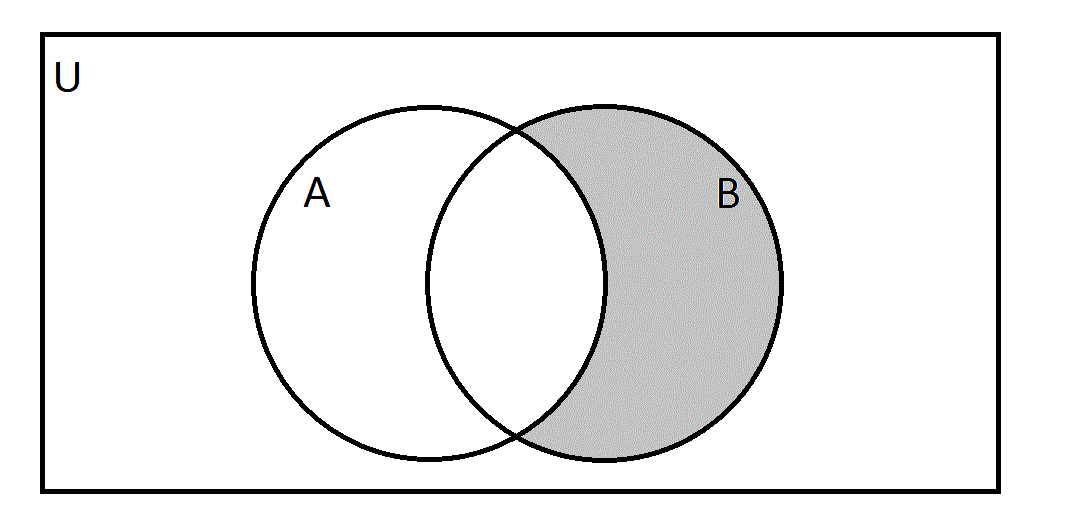Let set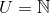, the set of all natural numbers.= {|is a multiple of 6 }= {|is a multiple of 9 }

Which of the following numbers would appear in the gray region of the Venn diagram?

Possible Answers: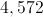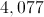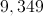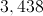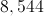Correct answer:Explanation:

The gray area represents the portion ofthat is not in- in other words, all multiples of 9 that are not also multiples of 6.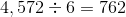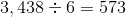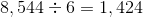Therefore, 4,572, 3,438, and 8,544 can be eliminated.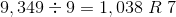, so 9,349 can be eliminated because it isn't a multiple of 9.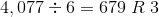and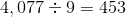, so, as both a nonmultiple of 6 and a multiple of 9, 4.077 is the correct choice.

### Example Question #2 : Data Analysis And Probability

If two events are independent of one another, there will be no intersection of their circles on a Venn Diagram.

Possible Answers:

True

False

Correct answer:

True

Explanation:

If the two events in a problem are independent, they cannot occur at the same time.  That means their circle will not intersect and they will not have a shared middle section.

### Example Question #1 : Range

On the last day of school,Adam asked each of his classmates how many days of school they missed for the entire school year.  He created a stem-and-leaf plot with the information.

0 | 0 0 2 4 5 6 8

1 | 0 0 1 2

2 | 3

4 | 7

What is the range of the number of days missed by Adam's classmates?

Possible Answers: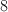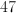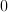Correct answer:Explanation:

To find the range of a set of data, simply subtract the smallest total from the largest total.  The left side of a stem-and-leaf plot represents the ten's digit, and the right side represents the one's digit. The largest value here is 47.  The smallest value is 0.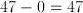### Example Question #4 : Data Analysis And Probability

Find the range in this set of numbers:

4520, 7325, 8732, 8321, 9031, 3117, 6960

Possible Answers: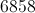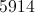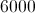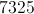Correct answer:Explanation:

First order the numbers from least to greatest: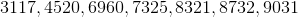Then find the difference of the greatest and the smallest number: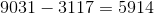Answer: The range is 5914.

### Example Question #5 : Data Analysis And Probability

Find the range in this set of numbers:

6059, 6609, 6906, 6509, 6905, 6960, 6950

Possible Answers: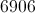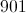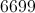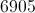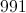Correct answer:Explanation:

First, order the numbers in the set from least to greatest: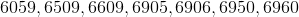Then, find the difference between the largest and the smallest number: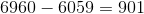Answer: The range is 901.

### Example Question #6 : Data Analysis And Probability

Find the range in this set of numbers:

640, 782, 411, 398, 299, 344

Possible Answers: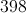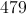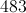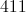Correct answer:Explanation:

First, order the numbers from least to greatest: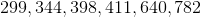Then, find the difference between the largest and smallest number: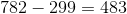Answer: The range is 483.

### Example Question #7 : Data Analysis And Probability

Find the range in this set of numbers:

9881, 9889, 9818, 9981, 9891, 9918, 9989, 9889, 9198

Possible Answers: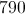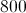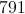Correct answer:Explanation:

First, order the numbers from least to greatest: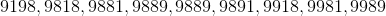Then, find the difference of the largest and smallest number: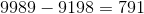Answer: The range is 791.

### Example Question #8 : Data Analysis And Probability

Find the range in this set of numbers:

3343, 4434, 4334, 3343, 4343, 3434, 3334

Possible Answers: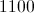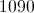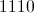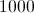Correct answer:Explanation:

First, order the numbers from least to greatest: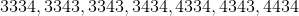Then, find the difference between the largest and the smallest number: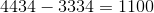Answer: The range is 1100.

### Example Question #9 : Data Analysis And Probability

Subtract the range from the mode in this set of numbers: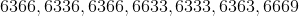Possible Answers: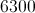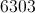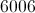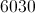Correct answer:Explanation:

First, order the numbers from least to greatest: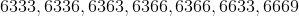Then, identify the mode (most recurring number): 6366

Then, identify the range (subtract the smallest number from the largest):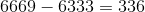Finally, subtract the range from the mode: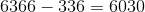Answer: 330

### Example Question #10 : Data Analysis And Probability

Find the range for this set of numbers:

6363, 6336, 6366, 6633, 6333, 6363, 6663

Possible Answers: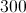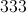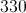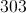Correct answer:Explanation:

First, order the numbers from least to greatest: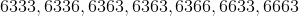Then, find the difference of the largest and the smallest numbers: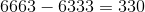Answer: The range is 330.

← Previous 1 3 4 5 6 7 8 9 57 58

### All ISEE Middle Level Math Resources